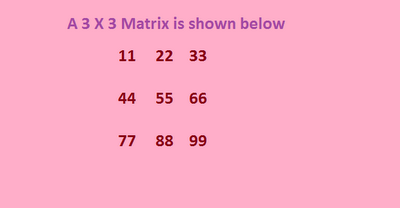## Wednesday, 12 December 2012

### Determinant of a Matrix using Recursion

A matrix is a rectangular array representation of numbers. Determinant exists only for square Matrices which have equal number of rows and columns. Here a recursive algorithm is used to find out the determinant of the given matrix.The Determinant of the given matrix is 0.